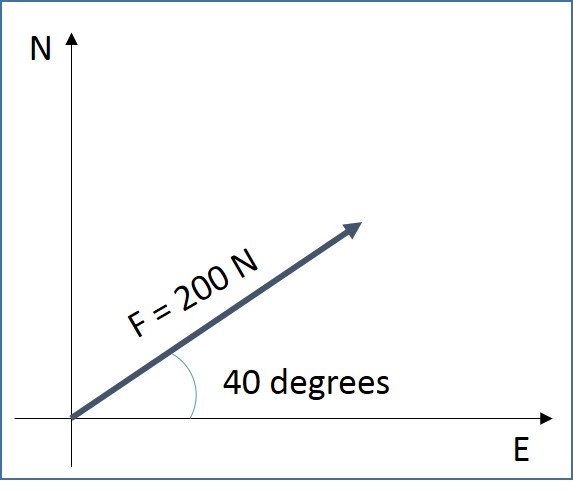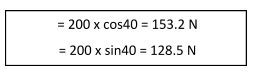# Scalars & Vectors

## Introduction

Consider a car that is travelling from city A to city B. The distance travelled by the car can be calculated by multiplying the average speed of the car and the time taken. However, we cannot find out how far the car is from its starting point unless we are told the direction of travel. Therefore, direction must be specified for some quantity.

A. Scalar Quantity

A quantity, which can be described fully by its magnitude, is defined as a scalar quantity. The mass of an object, speed of a car and pressure and temperature of a liquid are some examples of scalar quantity.

B. Vector Quantity

A quantity with magnitude and direction is defined as a vector quantity. The weight of an object, velocity and acceleration of a vehicle and the force acting on a bridge are some examples of vector quantity. One way to represent a vector is by means of an arrow. The direction of the arrow is in the direction of the vector quantity. The length of the arrow, drawn to scale, represents its magnitude. A force vector with quantity and direction is illustrated in Figure 1.Figure 1: Vector Illustration

• Resolution of vectors

Consider the force illustrated in Figure 1. The force vector at an angle of 400 can be split, or resolved, into two vectors, or components as illustrated in Figure 2. The combined effect of the components is the same as the original vector. The following equations will be used to resolve any vector quantity.Figure 2: Resolution of Vector

For the above example, a vector can be resolved as shown below.• Condition for Equilibrium

As illustrated in Figure 3, three forces (F1, F2 and F3) are in equilibrium when they form a closed triangle. In the below example, forces F1, F2 and F3 are drawn to their respective magnitude and angle.Figure 3: Forces in Equilibrium

C. Examples

The following table shows a few examples of scalar and vector quantities with sample values.D. Summary

• A quantity, which can be described fully by its magnitude, is defined as a scalar quantity.
• A quantity with magnitude and direction is defined as a vector quantity.
• Any vector can be resolved into horizontal and vertical components.
• Three forces (F1, F2 and F3) are in equilibrium when they form a closed triangle.